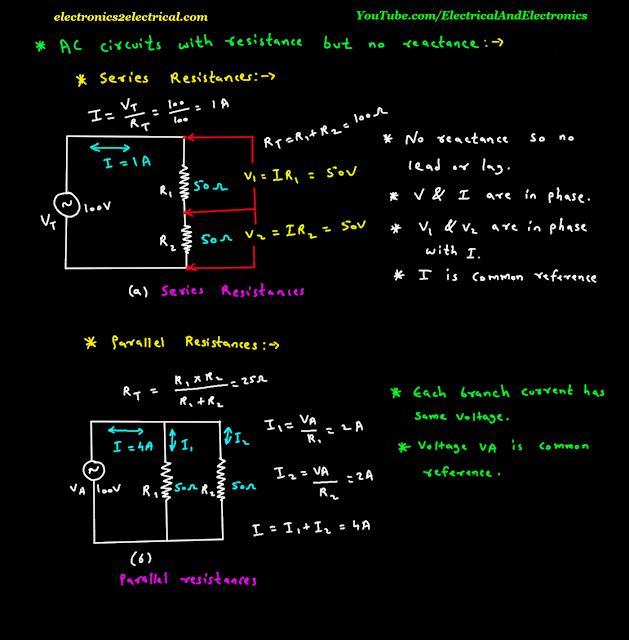AC Circuits with Resistance but No Reactance

0 like 0 dislike

AC Circuits with Resistance but No Reactance

AC Circuits with Resistance but No Reactance

Series resistances

let two resistances R 1 and R 2 are connected in series as shows in figure and there values are 50 ohm and 50 ohm respectively.

The AC voltage source is applied.

The value of AC voltage source is 100 volts.

The current flowing in the series circuit is calculated by total voltage V T upon Total resistance R T.

So the current flowing through the series circuit is 1 ampere.

Also according to ohm's law we can find the voltage across resistance R 1 and Resistance R 2.

Voltage across R 1 will be V 1 is equal to Current I into Resistance R 1.

Voltage across R 2 will be V 2 is equal to current I into resistance R 2.

The total resistance will be R T is equal to R 1 plus R 2.

Since there is no reactance in the circuit there will be no lead or lag in the voltage or current.

In resistive circuit voltage and current are in phase.

Also voltage V 1 and V 2 are in phase with current I.

Since the current flowing through both the resistance is same the common reference will be current I.Now parallel resistances

Let the two resistances are connected in parallel.

They have same 50 ohm and 50 ohm values and 100 volts AC voltage source.

The total resistance R T can be find by R 1 into R 2 upon R 1 plus R 2.

Both the branch are connected in parallel so they have same voltage across them.

The current I in resistance R 1 and R 2 can be find by Ohm's law.

I 1 is equal to Voltage V A upon resistance R 1.

I 2 is equal to voltage V A upon resistance R 2.

Total current is equal to current I 1 plus current I 2.

Each branch current has same voltage.

Voltage V A is common to both the branch hence voltage V A is common reference.